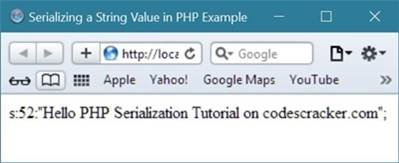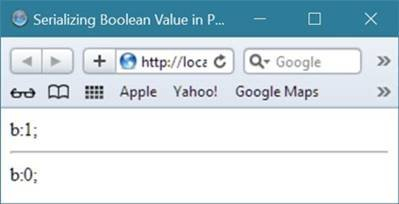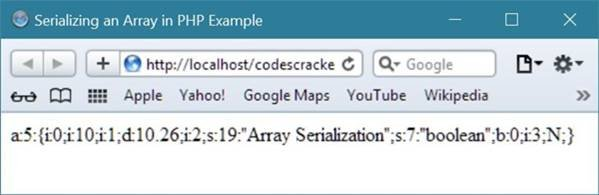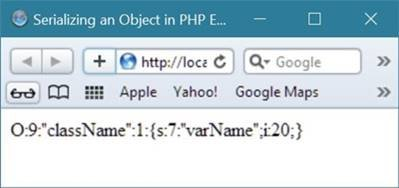# PHP Serialization

In PHP, serialization is used to store or pass any PHP values around without losing the value's type and structure.

Basically, serialization in PHP generates a storable representation of a PHP value.

## PHP serialize() function

The PHP serialize() function is used to serialize any PHP value.

## PHP Serialization Example

Let's see some examples given below, of serialization in PHP for different-different PHP type.

## Serializing String in PHP

Here is an example demonstrates about serializing a string in PHP.

```<html>
<title>Serializing a String Value in PHP Example</title>
<body>
<?php
\$original_string = "Hello PHP Serialization Tutorial on codescracker.com";
\$serialize_string = serialize(\$original_string);
echo \$serialize_string;
?>
</body>
</html>```

Here is the sample output that will be shown in your browser after running the above PHP example of serializing a string value.## Serializing Double in PHP

Below is an example of serializing double in PHP.

```<html>
<title>Serializing a Double Value in PHP Example</title>
<body>
<?php
\$original_value = 10.65;
\$serialized_value = serialize(\$original_value);
echo \$serialized_value;
?>
</body>
</html>```

Below is the sample output of above serializing double in PHP example code:In the same way, you can serialize both Float and Integer value in PHP. Now let's serialize boolean value in PHP using serialize() function.

## Serializing Boolean in PHP

Below is an example of serializing boolean value in PHP.

```<html>
<title>Serializing Boolean Value in PHP Example</title>
<body>
<?php
// let's first check for true (boolean value)
\$orig_bool_val = true;
\$serialize_bool_val = serialize(\$orig_bool_val);
echo \$serialize_bool_val;

echo "<hr/>";

// now let's check for false (boolean value)
\$orig_bool_val = false;
\$serialize_bool_val = serialize(\$orig_bool_val);
echo \$serialize_bool_val;
?>
</body>
</html>```

Below is the sample output produced by the above serializing boolean value example code in PHP.## Serializing Null in PHP

Let's serialize null value in PHP using the following example code:

```<html>
<title>Serializing Null in PHP Example</title>
<body>
<?php
\$orig_null_val = null;
\$serialize_null_val = serialize(\$orig_null_val);
echo \$serialize_null_val;
?>
</body>
</html>```

Following is the sample output of the above example code of serializing null in PHP.## Serializing Array in PHP

Let's serialize an array in PHP with the help of following example code:

```<html>
<title>Serializing an Array in PHP Example</title>
<body>
<?php
\$orig_array_val = array(
10,
10.26,
'Array Serialization',
'boolean'=>false,
null
);
\$serialize_array_val = serialize(\$orig_array_val);
echo \$serialize_array_val;
?>
</body>
</html>```

Here is the sample output of the above serializing an array value in PHP example code:## Serializing Object in PHP

Now let's serialize an object in PHP using the following example code:

```<html>
<title>Serializing an Object in PHP Example</title>
<body>
<?php
class className
{
var \$varName = 20;
function functionName()
{
return 'return value';
}
}
\$orig_object = new className();
\$serialize_object = serialize(\$orig_object);
echo \$serialize_object;
?>
</body>
</html>```

Below is the sample output produce by the above example code of serializing an object in PHP.Tools
Calculator